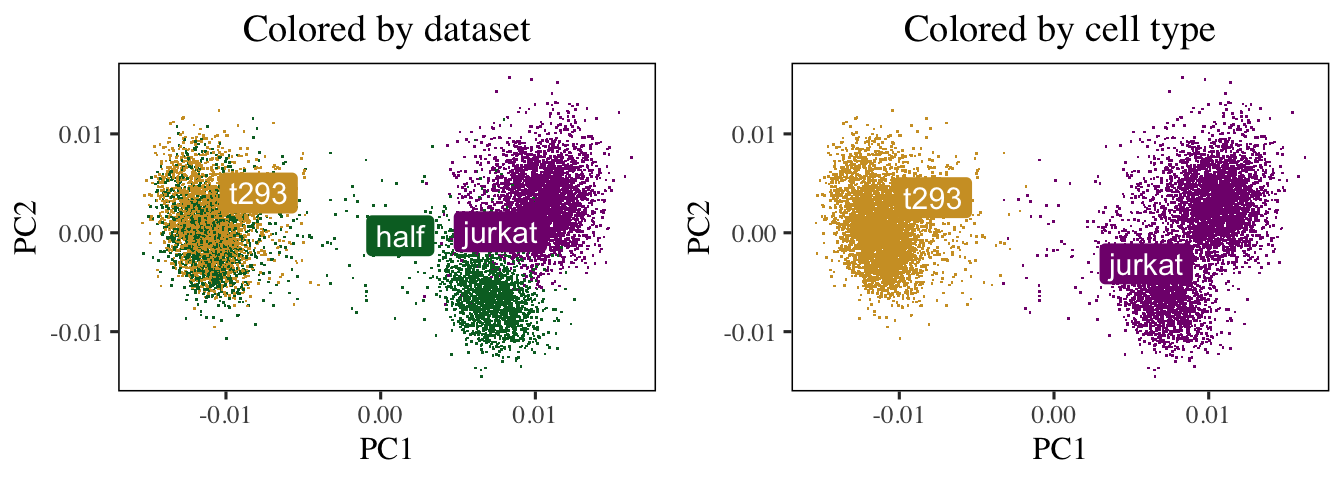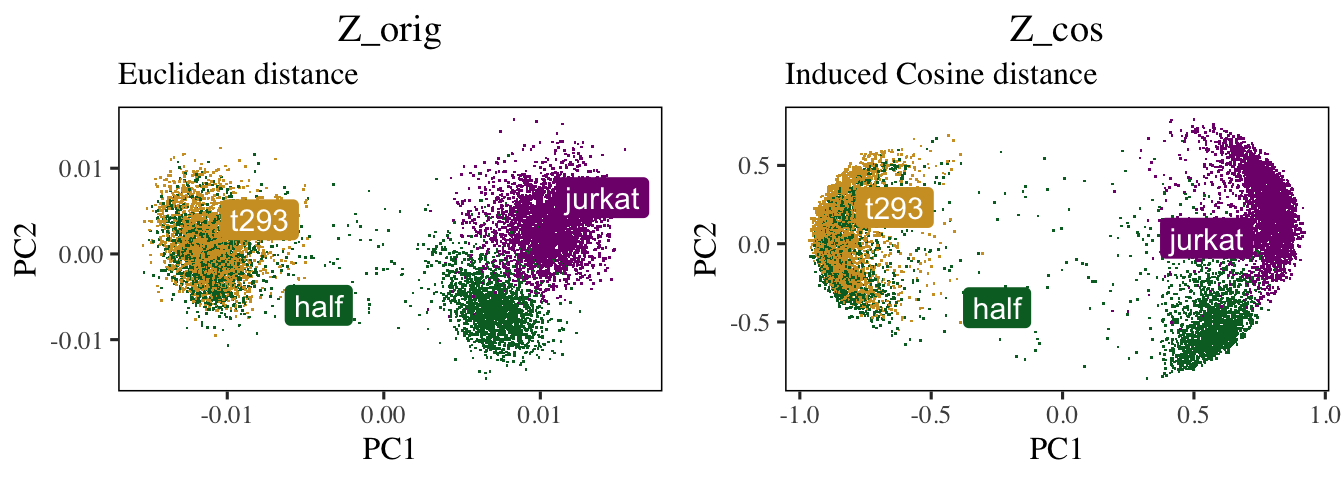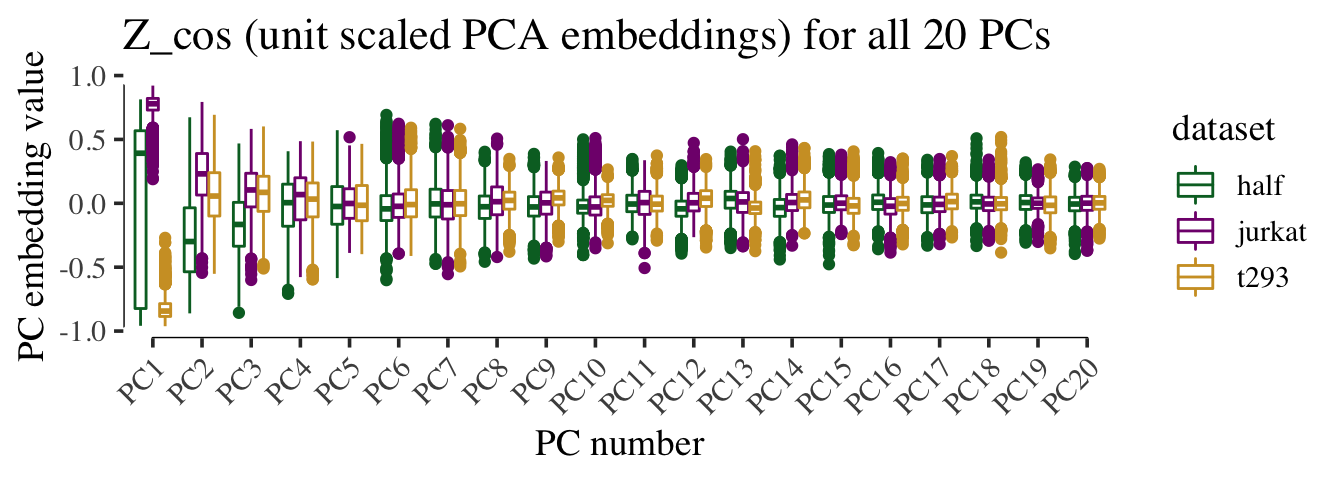# 1 Motivation

This notebook breaks down the Harmony algorithm and model in the context of a simple real-world dataset.

After reading this, the user should have a better understanding of how

1. the equations connect to the algorithm
2. the algorithm works on real data
3. to access the different parts of the Harmony model from R

# 2 Cell line data

This dataset is described in figure 2 of the Harmony manuscript. We downloaded 3 cell line datasets from the 10X website. The first two (jurkat and 293t) come from pure cell lines while the half dataset is a 50:50 mixture of Jurkat and HEK293T cells. We inferred cell type with the canonical marker XIST, since the two cell lines come from 1 male and 1 female donor.

We library normalized the cells, log transformed the counts, and scaled the genes. Then we performed PCA and kept the top 20 PCs. We begin the analysis in this notebook from here.

V <- harmony::cell_lines$scaled_pcs V_cos <- cosine_normalize(V, 1) meta_data <- harmony::cell_lines$meta_data

To get a feel for the data, let’s visualize the cells in PCA space. The plots below show the cells’ PC1 and PC2 embeddings. We color the cells by dataset of origin (left) and cell type (right).

do_scatter(V, meta_data, 'dataset', no_guides = TRUE, do_labels = TRUE) +
labs(title = 'Colored by dataset', x = 'PC1', y = 'PC2') +
do_scatter(V, meta_data, 'cell_type', no_guides = TRUE, do_labels = TRUE) +
labs(title = 'Colored by cell type', x = 'PC1', y = 'PC2') +
NULL# 3 Initialize a Harmony object

The first thing we do is initialize a Harmony object. We pass 2 data structures:

1. V: the PCA embedding matrix of cells.
2. meta_data: a dataframe object containing the variables we’d like to Harmonize over.

The rest of the parameters are described below. A few notes:

• nclust in the R code below corresponds to the parameter K in the manuscript.
• we set max.iter.harmony to 0 because in this tutorial, we don’t want to actually run Harmony just yet.
• setting return_object to TRUE means that harmonyObj below is not a corrected PCA embeddings matrix. Instead, it is the full Harmony model object. We’ll have a closer look into the different pieces of this object as we go!
set.seed(1)
harmonyObj <- harmony::HarmonyMatrix(
data_mat = V, ## PCA embedding matrix of cells
meta_data = meta_data, ## dataframe with cell labels
theta = 1, ## cluster diversity enforcement
vars_use = 'dataset', ## variable to integrate out
nclust = 5, ## number of clusters in Harmony model
max.iter.harmony = 0, ## stop after initialization
return_object = TRUE, ## return the full Harmony model object
do_pca = FALSE ## don't recompute PCs
)

By initializing the object, we have prepared the data in 2 ways. First, we’ve scaled the PCA matrix to give each cell unit length. Second, we’ve initialized cluster centroids with regular kmeans clustering on these scaled data. We’ll dig into these two steps below.

## 3.1 L_2 scaling to induce cosine distance

A key preprocessing step of Harmony clustering is L2 normalization. As shown in Haghverdi et al 2018, scaling each cell to have L2 norm equal to 1 induces a special property: Euclidean distance of the scaled cells is equivalent to cosine distance of the unscaled cells. Cosine distance is a considerably more robust measure of cell-to-cell similarity (CITE Martin and Vlad). Moreover, it has been used in clustering analysis of high dimensional text datasets (CITE NLP spherical kmeans).

$$L_2$$ Normalization of cell $$i$$:

$$\hat{Z}_{\cdot, i} \leftarrow \frac{\hat{Z}_{\cdot, i}}{||{\hat{Z}_{\cdot, i}}||_{2}}$$

TL;DR Harmony clustering uses cosine distance. By normalizing each cell to have unit length, we can directly visualize the cosine distances between cells (right). These relationships are not obvious in Euclidean space (left).

In the Harmony object, we now have 2 copies of the cell embeddings. The first, $$Z_{orig}$$ is the original PCA matrix (PCs by cells). The second, $$Z_{cos}$$ is the new $$L_2$$ scaled matrix. Since this scaling projects cells into a unit hypersphere, cells appear pushed away from the origin (0,0).

do_scatter(t(harmonyObj$Z_orig), meta_data, 'dataset', no_guides = TRUE, do_labels = TRUE) + labs(title = 'Z_orig', subtitle = 'Euclidean distance', x = 'PC1', y = 'PC2') + do_scatter(t(harmonyObj$Z_cos), meta_data, 'dataset', no_guides = TRUE, do_labels = TRUE) +
labs(title = 'Z_cos', subtitle = 'Induced Cosine distance', x = 'PC1', y = 'PC2') +
NULLIn the $$Z_{cos}$$ scatterplot (right), cells that are nearby have a high cosine similarity. Although it is not obvious in this example, cells closeby in Euclidean space do not always have a high cosine similarity!

Above, we only visualize the first two PCs. In this simple example with cell lines, this is sufficient to visualize most of the variation. Note, however, that all clustering and correction in Harmony uses all the PCs. For completeness, we can visualize the quantiles of PCA embeddings for all 20 PCs, colored by original dataset.

harmonyObj$Z_cos %>% t %>% data.frame() %>% cbind(meta_data) %>% tidyr::gather(key, val, X1:X20) %>% ggplot(aes(reorder(gsub('X', 'PC', key), as.integer(gsub('X', '', key))), val)) + geom_boxplot(aes(color = dataset)) + scale_color_manual(values = colors_use) + labs(x = 'PC number', y = 'PC embedding value', title = 'Z_cos (unit scaled PCA embeddings) for all 20 PCs') + theme_tufte(base_size = 14) + geom_rangeframe() + theme(axis.text.x = element_text(angle = 45, hjust = 1)) + NULL## 3.2 Initial clustering Initializing the Harmony object also triggered initialization of all the clustering data structures. Harmony currently uses regular kmeans, with 10 random restarts, to find initial locations for the cluster centroids. Let’s visualize these centroids directly! We can do this by accessing the Y matrix in the Harmony object. This is a matrix with $$d=20$$ rows and $$K=5$$ columns, so each column represents one 20-dimensional centroid. Remember that we set the number of clusters to 5 above, so there are now 5 clusters below. cluster_centroids <- harmonyObj$Y

do_scatter(t(harmonyObj\$Z_cos), meta_data, 'dataset', no_guides = FALSE, do_labels = FALSE) +
labs(title = 'Initial kmeans cluster centroids', subtitle = '', x = 'PC1', y = 'PC2') +
geom_point(
data = data.frame(t(cluster_centroids)),
color = 'black', fill = 'black', alpha = .8,
shape = 21, size = 6
) +
NULL